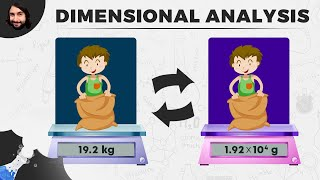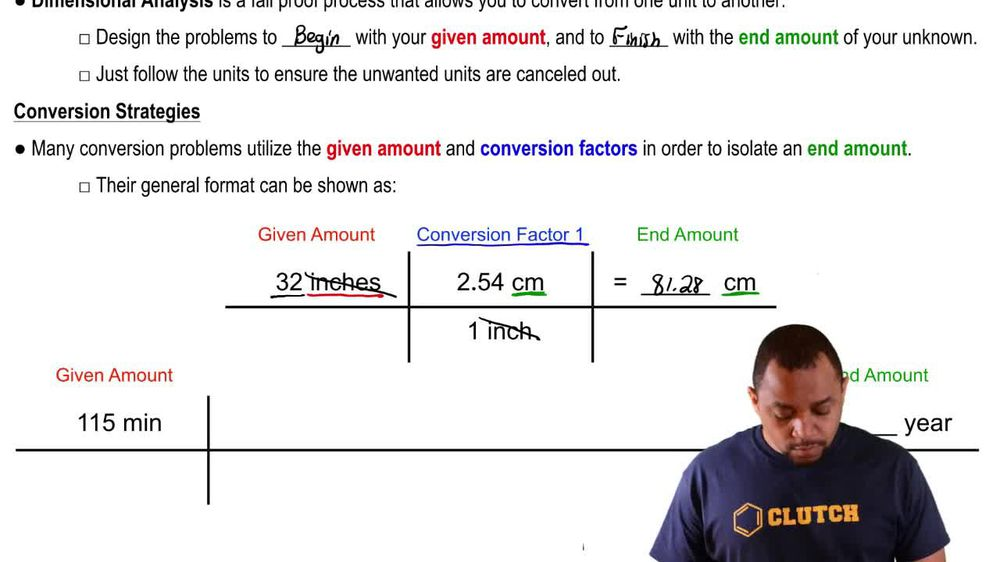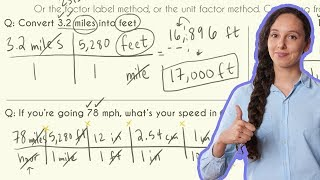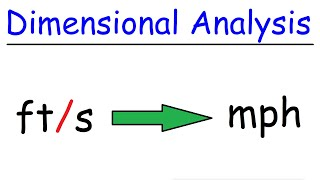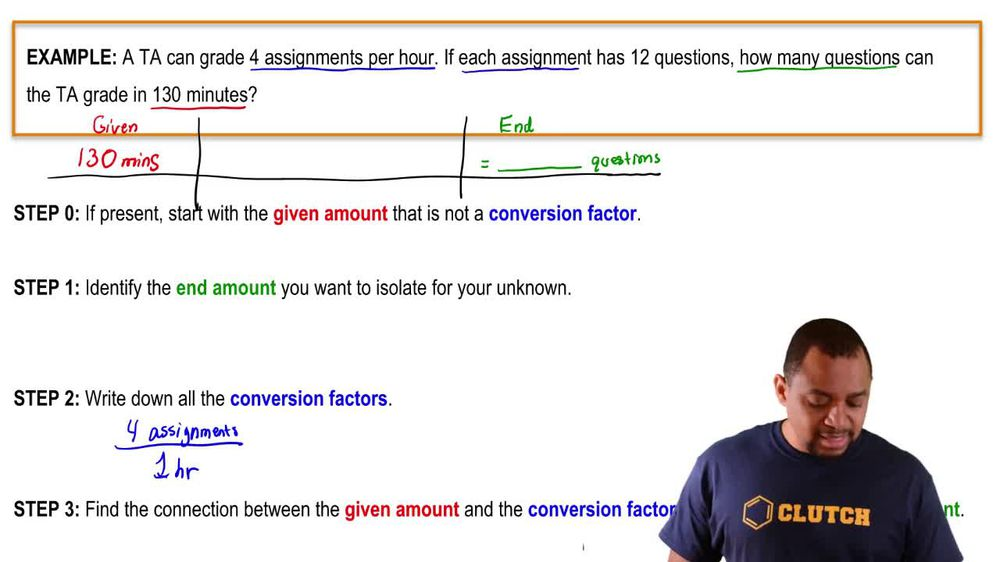Start typing, then use the up and down arrows to select an option from the list.
1. 1. Intro to General Chemistry2. Dimensional Analysis
Problem

# The total rate at which power is used by humans worldwide is approximately 15 TW (terawatts). The solar flux averaged over the sunlit half of Earth is 680 W>m2 (assuming no clouds). The area of Earth’s disc as seen from the Sun is 1.28 * 1014 m2. The surface area of Earth is approximately 197,000,000 square miles. How much of Earth’s surface would we need to cover with solar energy collectors to power the planet for use by all humans? Assume that the solar energy collectors can convert only 10% of the available sunlight into useful power

Relevant Solution3m
Play a video:
Hey everyone. So here we're told that solar power is produced from sunlight striking earth and it says that it strikes the earth, which is approximately 186 million square miles in terms of its area at a rate of 1.447 kilowatts per square meter. Now here we're told that solar energy collectors can convert 10% of it into useful power. Now, if humans use a total of 17 terra watts, what percentage of earth should be covered with solar energy collectors to provide sufficient energy or power. Alright, so we have this amount as our kilowatts per square meter, But only 10% of it is being converted into useful power. So we can't use percentages. We need to use the decimal form. So we divide our percentage by 100. That gives me .10. Then what I do is I take my amount of energy and I multiplied by the .10. This is the amount of useful energy we can use now that we know that. Let's see how much land would we need if human beings themselves need 17 terra wants. So we're going to take the 17 terra wants. That's given to us within the question We're gonna say 17 terra watts. We're gonna convert from terra wants to wants. Remember that one terror is equal to 10 to the 12th And that one kg is 10 to the three. Now that we have kilowatts we can bring down the useful power. So that's 0.1447 kilowatts per one m squared. This tells me that I need 1.1748 times 10 to the m squared of land. Now, within the question, we're told this is the amount of square miles the earth encompasses. So we're gonna convert that into scientific notation, which is 1.86 times 10 to the eight miles squared here. We need to convert the mile squared into meters squared so that it matches these units we got earlier. So we're gonna stay here. one km is equal 2.621371 mi. Since the miles are squared, I'm gonna have to square this portion as well. And then we're going to say next. Since I have km. Now, I'm going to say that one km is equal 10-3 m. And this is also squared Doing that gives me 4.815 times 10 to the 14 m squared. So I have the amount of land I need for my energy collectors and I have the amount of area of the entire earth. So I'm gonna take those two numbers. I'm gonna 1.174, 8 Times 10 to the 11 m squared, Divided by 4.8, 1, 5 times 10 to the 14 m squared. I'm gonna multiply that by a 100 And that tells me I'm going to need .02 Of the Earth's surface to supply 17 TRA wants to humans. So really it's not that much space that we would need to provide sufficient energy for humans based on these numbers given within this question.